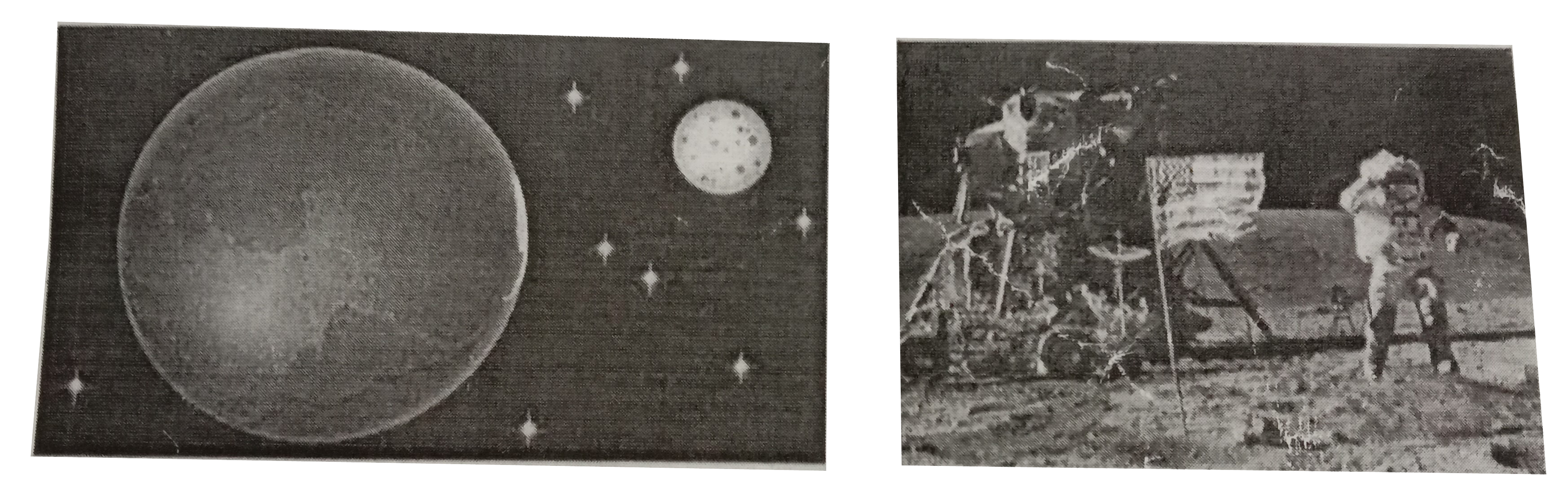Question

Updated on:30/05/2023

# Calculate the value of acceleration due to gravity on moon. Given mass of moon =7.4×1022 kg, radius of moon=1740 km.Please note that the value of g on the moon is about one-sixth (1/6) of the value of g on the earth (the value of acceleration due to gravity on the earth being 9.8 m/s2